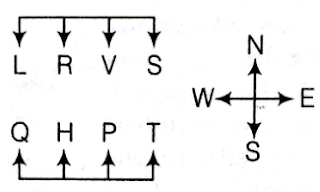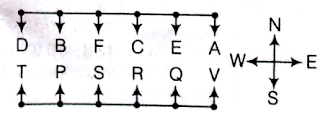### Reasoning- Sitting Arrangement Quiz

Directions (1-5): Study the following information carefully to answer these questions.

Eight members P, Q, R, S, T, V, H and L are sitting in rows with equal number of members in each row. Members of one row are facing north and those in the other row is sitting exactly opposite a member in the other row.
P sits in the row facing north, to the immediate right of H who is exactly opposite of R. L is to the immediate right of R and S is exactly opposite of T who is to the immediate right of P. V does not sit at any of the ends of any row.

1). Which of the following members sit at the ends of the same row?
a) TH
b) SR
c) LQ
d) LT
e) None of these

2). Who sits opposite of P?
a) L
b) Q
c) V
d) Cannot be determined
e) None of these

3). Which of the following members sit exactly opposite each other?
a) VQ
b) LV
c) VH
d) VP
e) None of these

4). Who is to the left of V?
a) T
b) S
c) R
d) L
e) None of these

5). Who sits to the right of Q?
a) H
b) L
c) V
d) R
e) None of these

Directions (Q. 6-12): Study the following information carefully to answer these questions.

Twelve people are sitting in two parallel rows containing six people each, in such a way that there is an equal distance between adjacent persons. In row 1, A, B, C, D, E, and F are seated and all of them are facing south. In row 2, P. Q. R, S, T and V are seated and all of them facing north. Therefore in the given seating arrangement each member seated in a row faces another member of the other row.
V sits third to the right of S. S faces F and F does not sit at any of the extreme ands of the line. D sits third to right of C. R faces C. The one facing E sits third to right of P. B and B do not sit at the extreme ends of the line. T is not an immediate neighbor of V and A is not an immediate neighbor of C.

6). Who amongst the following faces D?
a) T
b) P
c) Q
d) R
e) None of these

7). Who amongst the following represent the people sitting at extreme ends of the rows?
a) R, F
b) T, A
c) D, R
d) C, Q
e) S, A

8). Four of the following five are alike in a certain way and thus from a group. Which is the one that does not belong to that group?
a) B-T
b) A-Q
c) C-S
d) F-P
e) D-R

9). Four of the following five are alike in a certain way and thus from a group. Which is the one that does not belong to that group?
a) D
b) S
c) V
d) T
e) A

10). How many persons are seated between R and T?
a) One
b) Two
c) Three
d) Four
e) None of these

11). Who amongst the following faces Q?
a) D
b) B
c) E
d) A
e) None of these

12). Who amongst the following is true regarding?
a) B sits to the immediate left of C
b) B faces Q
c) B sits fourth from the extreme left end of the line
d) D and F are immediate neighbors of B
e) None is true

1) e)
2) c)
3) d)
4) b)
5) a)
6) a)
7) b)
8) e)
9) b)
10) b)
11) c)
12) d)

Solutions:
Questions (1-5):

According to given information, the final arrangement is as follows1. LS and QT sit at the ends of same row

2. V sits opposite of P.

3. V and P sit exactly opposite to each other.

4. S is to the left of V.

5. H sits to the right of Q.

Questions (6-12):

According to information, the final arrangement is as follows6. T faces D

7. T and A sit at extreme ends of the row.

8. Except D-R, all others are diagonally opposite to each other.

9. Except S, all other are sitting at extreme ends of the rows.

10. Two persons (P and S) are sitting between R and T.